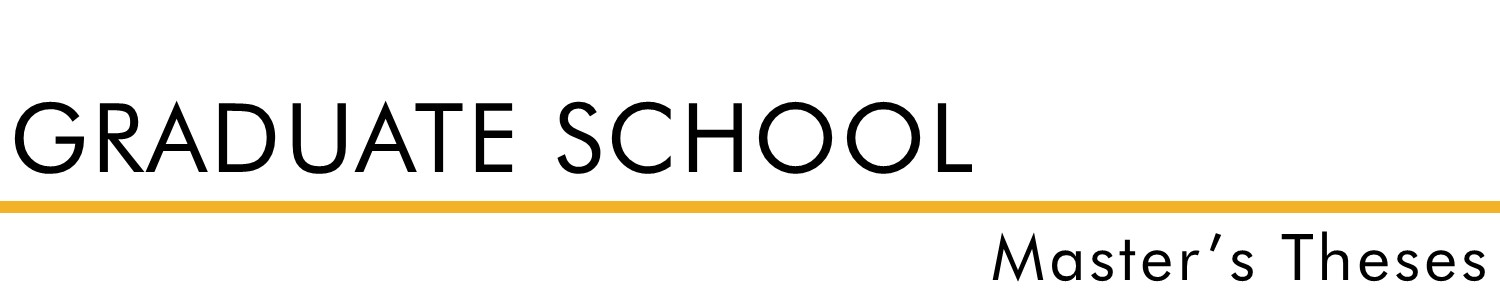#### Title

A Study of Pell's Equation

Spring 1968

#### Degree Name

Master of Arts (MA)

Math

Wilmont Toalson

#### Abstract

The purpose of this paper is to develop a method for finding values of N for which the equation x[squared] - DY[squared] = N will have integral solution in terms of X and Y for a given value of D which is a natural number, not a perfect square. It is shown that values of N are determined by the particular form of D. Results are summarized in the following list where c and k are natural numbers and the various forms of D are paired with the corresponding values of N. [See manuscript for list]

#### Rights

Copyright 1968 W.H. Lewis